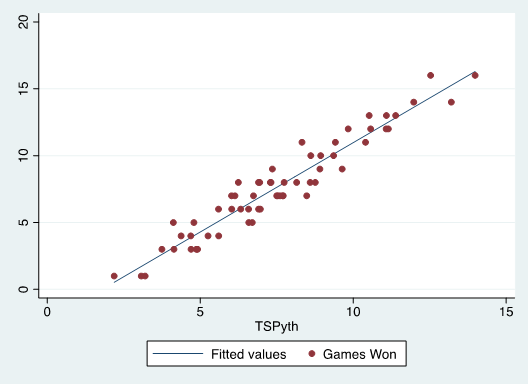# Bringing Pythagorean Expectation to College Lacrosse

By Austin Tymins

The Pythagorean expectation is a predictive win model originally developed for use in baseball that uses a formula similar to the Pythagorean theorem using runs scored and runs allowed to predict winning percentage. The calculated Pythagorean winning percentage can therefore be used to evaluate how lucky a team is at the end of the season by examining the difference between the true winning percentage and the expected one. The expectation can also be used early in a season to approximate a team’s winning percentage at the end of the season.

A typical Pythagorean expectation formula is of this form below where e is a calculated exponent that varies from sport to sport.

Expected Wins=Games Played*Goals Scored^(e)/(Goals Scored^(e)+Goals Allowed^(e))

This family of formulas is applicable across sports with varying e values. The exponent used in traditional Pythagorean formulas by league is:

• EPL : 1.3
• NHL : 2.15
• NFL : 2.37
• NBA : 13.91

To find the lacrosse-specific exponent, I took the difference between each team’s actual win total and their expected win total and squared that amount. After doing the same process for each team in lacrosse I added the values together. Taking the square root of this finally gives us the root-mean-square error for the league. To find the best exponent, I began looking for the exponent that would minimize the league RMSE, or the least error between my expected win percentage and the actual one.

Using the method I described and by looking at the 2012 and 2013 seasons, I found the exponent with the best fit to be 3.12. My expected wins model using Pythagorean expectation is now:

Expected Wins=Games Played*Goals Scored^(3.12)/(Goals Scored^(3.12)+Goals Allowed^(3.12))

I then used the 3.12 league average exponent to determine a team-specific exponent that further extends the predictive ability of the model. And by doing this, I no longer have to reduce the exponent to a single number for any team. This approach, first used in baseball, is known as the Smyth/Patriot method (Pythagenpat) and is used to find a team-specific exponent in a new formula:

Team Exponent=(Goals + Goals Allowed/Games Played)^e

And optimally: e=.376

After adding the team-specific exponent to the model, I performed a standardized residual test to remove three outlying points. After doing this, I tested for heteroskedasticity, nonlinearity, and normality of error terms. My model passed all three tests with flying colors and yielded a final R-squared= 0.9228 and Root MSE= 1.0195.For a future post, I would like to add “second-order wins” to my model, which currently only looks at first-order ones. First-order wins are purely based on goal differential, second-order would better account for luck by looking at expected goals scored and allowed in place of actual goals scored and allowed.

Additionally, the application of Bill James’s log5 formula from baseball, or something similar, would be an interesting application of this model to predict postseason matches. In this way, my model could very easily be used to calculate win probabilities in matchups between any teams.